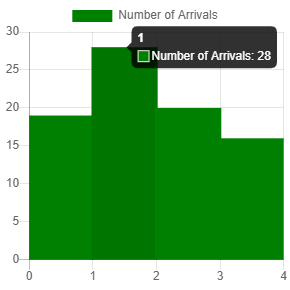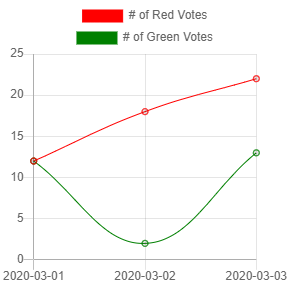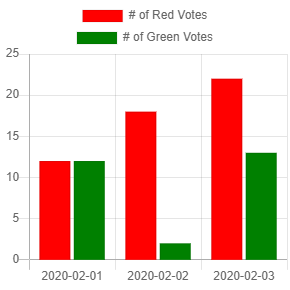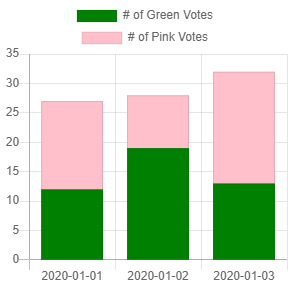Categories

## Chart.js – Create a Histogram

We can create a histogram with Chart.js without a problem.

All we have to do is to create a bar chart with some tweaks.

To start, we add Chart.js for the chart and the canvas for Chart.js to render the chart.

We add them by writing the following HTML:

``````<script src='https://cdnjs.cloudflare.com/ajax/libs/Chart.js/2.9.3/Chart.min.js'></script>

<canvas id="histogram" width="400" height="400"></canvas>
``````

Next, we create our histogram by writing some JavaScript code:

``````const ctx = document.getElementById('histogram').getContext('2d');

const chart = new Chart(ctx, {
type: 'bar',
data: {
labels: [0, 1, 2, 3, 4],
datasets: [{
label: 'Number of Arrivals',
data: [19, 28, 20, 16],
backgroundColor: 'green',
}]
},
options: {
scales: {
xAxes: [{
display: false,
barPercentage: 1.3,
ticks: {
max: 3,
}
}, {
display: true,
ticks: {
autoSkip: false,
max: 4,
}
}],
yAxes: [{
ticks: {
beginAtZero: true
}
}]
}
}
});
``````

We render the chart with the canvas element with the ID histogram as we indicated in the code.

In the object that we passed into the `Chart` constructor, we set the `type` to `'bar'` to display a bar chart.

Then in `datasets`, we add our `data` property to set the bar heights.

The `labels` are the labels that are displayed on the x-axis.

`label` is displayed above the histogram.

Then we have to adjust the x-axis options by setting `ticks` maximum to 4 to adjust the ticks.

We make the bars wider than the typical graph by setting `barPercentage` to 1.3 so that the 4 bars are event distributed between the 5 ticks of the x-axis.

`autoSkip` set to `false` means that automatic calculation of how many labels can be shown or hidden is false.

This lets us override the ticks with the ones that we mentioned above.

`autoSkip` being `false` means that we always show all labels.

`beginAtZero` being `true` means that we start the y-axis at 0.

In the end, we get the following graph:We can see the green bars and the bar is between 2 ticks rather than the bar being right above each tick.

Categories

## Chart.js – Creating a Chart with Multiple Lines

To create a chart with multiple lines, we can just create a line chart that display multiple data sets.

Also, we add the moment.js library for formatting dates, and a canvas element for Chart.js to render the chart in.

We do that by writing:

``````<script src='https://cdnjs.cloudflare.com/ajax/libs/Chart.js/2.9.3/Chart.min.js'></script>
<script src='https://cdnjs.cloudflare.com/ajax/libs/moment.js/2.24.0/moment.min.js'></script>

<canvas id="multipleLineChart" width="400" height="400"></canvas>
``````

Next, we create our chart with multiple lines by writing the following:

``````const ctx = document.getElementById('multipleLineChart').getContext('2d');

const chart = new Chart(ctx, {
type: 'line',
data: {
labels: [
moment(new Date(2020, 2, 1)).format('YYYY-MM-DD'),
moment(new Date(2020, 2, 2)).format('YYYY-MM-DD'),
moment(new Date(2020, 2, 3)).format('YYYY-MM-DD')
],
datasets: [{
data: [12, 18, 22],
borderWidth: 1,
fill: false,
borderColor: 'red'
},
{
data: [12, 2, 13],
borderWidth: 1,
fill: false,
borderColor: 'green'
}
]
},
options: {
scales: {
yAxes: [{
ticks: {
beginAtZero: true
}
}]
}
}
});
``````

We set the `type` property to `'line'` to display line charts.

Then we have the `labels` property to display the labels for the x-axis.

In the `datasets` property, we set the value to an array.

The array has the `data` property to set the y-axis value for where the dot is displayed.

We set the `fill` property to `false` so that we don’t get any filling between the line and the x-axis.

`borderColor` has the color value of the line. We set one to `'red'` and the other to `'green'`.

In the `options` property, we set the `beginAtZero` property to `true` so that the y-axis begins at zero.

Once we write that code, we get a chart with multiple lines with one being red and the other being green.

It looks like:Categories

## Create a Grouped Bar Chart with Chart.js

We can make a grouped bar chart with Chart.js by creating a bar chart that has multiple `datasets` entries.

To start, we first include the Chart.js library. We also include the moment.js library for formatting dates.

The grouped bar chart will be rendered in a canvas element.

So we write the following code to include all that:

``````<script src='https://cdnjs.cloudflare.com/ajax/libs/moment.js/2.24.0/moment.min.js'></script>

<canvas id="barChart" width="400" height="400"></canvas>
``````

Next, we add the code for rendering the grouped bar chart.

We write the following:

``````const ctx = document.getElementById('barChart').getContext('2d');

const chart = new Chart(ctx, {
type: 'bar',
data: {
labels: [
moment(new Date(2020, 1, 1)).format('YYYY-MM-DD'),
moment(new Date(2020, 1, 2)).format('YYYY-MM-DD'),
moment(new Date(2020, 1, 3)).format('YYYY-MM-DD')
],
datasets: [{
data: [12, 18, 22],
borderWidth: 1,
backgroundColor: ['red', 'red', 'red']
},
{
data: [12, 2, 13],
borderWidth: 1,
backgroundColor: ['green', 'green', 'green']
}
]
},
options: {
scales: {
yAxes: [{
ticks: {
beginAtZero: true
}
}]
}
}
});
``````

The code above has the `labels` property with the labels for the x-axis.

The `datasets` property has the data sets we display on the y-axis.

`data` has the bar heights for each bar.

`label` has the label for each bar.

`backgroundColor` has the background color for each bar.

`borderWidth` has the border width for each bar.

Then in the `options` property, we have the `beginAtZero` property to make sure that the y-axis starts at zero instead of the value of the lowest bar value.

In the end, we have:It’s a grouped bar chart with red and green bars displaying the data in the `data` arrays.

With Chart.js, creating a grouped bar chart is just a matter of setting the labels, bar heights, and bar colors of each bar.

Categories

## Create a Stack Bar Chart with Chart.js

We can create stacked bar chart with Chart.js

With the `stacked` option in Chart.js, we can create a stacked bar chart from a simple bar chart.

To create the chart, first we need to add Chart.js to our code by writing:

``````<script src='https://cdnjs.cloudflare.com/ajax/libs/Chart.js/2.9.3/Chart.min.js'></script>
<script src='https://cdnjs.cloudflare.com/ajax/libs/moment.js/2.24.0/moment.min.js'></script>

<canvas id="barChart" width="400" height="400"></canvas>
``````

The code above also adds moment.js for manipulating time and a canvas element to display the chart.

Then we write:

``````const ctx = document.getElementById('barChart').getContext('2d');

const chart = new Chart(ctx, {
type: 'bar',
data: {
labels: [
moment(new Date(2020, 0, 1)).format('YYYY-MM-DD'),
moment(new Date(2020, 0, 2)).format('YYYY-MM-DD'),
moment(new Date(2020, 0, 3)).format('YYYY-MM-DD')
],
datasets: [{
data: [12, 19, 13],
borderWidth: 1,
backgroundColor: ['green', 'green', 'green']
},
{
data: [15, 9, 19],
borderWidth: 1,
backgroundColor: ['pink', 'pink', 'pink']
}
]
},
options: {
scales: {
xAxes: [{
stacked: true
}],
yAxes: [{
stacked: true
}]
}
}
});
``````

We’ve specified by the labels in the `labels` property to be 3 dates.

In the `datasets` property, we have the `label` to display the label of the legtnd.

The `borderWidth` has the border with value of the bar in pixels.

The `backgroundColor` has the background color of the bars.

The `data` has the bar heights of the bars.

The `options` object has the property that lets us create a stacked bar chart.

We have the `xAxes` property, which has an array with an object that has `stacked` set to `true`.

Also, we have the same property in the object in the `yAxes` array.

Once we have that, we get:Creating a stacked bar chart is just as easy as add the data to display for each bar.

Then we set the `stacked` option in the `options` object to make 2 or more bars stacked together.

Categories

## Chart.js Bar Chart Example

Creating a bar chart isn’t very hard with Chart.js.

In this article, we’ll look at a Chart.js bar chart example to see how we can create a simple bar chart with little effort.

First, we have to include the Chart.js library. It uses the canvas to render chart data.

So, we have to write:

``````<script src='https://cdnjs.cloudflare.com/ajax/libs/Chart.js/2.9.3/Chart.min.js'></script>

<canvas id="barChart" width="400" height="400"></canvas>
``````

To add Chart.js and our canvas.

Then we can write the following JavaScript code to create our bar chart:

``````const ctx = document.getElementById('barChart').getContext('2d');

const colorLabels = ['red', 'green', 'blue', 'yellow', 'orange']

const chart = new Chart(ctx, {
type: 'bar',
data: {
labels: colorLabels,
datasets: [{
data: [12, 19, 3, 5, 2],
borderWidth: 1,
backgroundColor: colorLabels
}]
},
options: {
scales: {
yAxes: [{
ticks: {
beginAtZero: true
}
}]
}
}
});
``````

We set the `labels` property to set the labels at the x-axis.

The `data` array has the bar heights.

`borderWidth` sets the border widths of the bars.

`backgroundColor` set the colors of the bars.

In the `options` property, we have the `yAxes` property, which is an array.

In the array entry, we have the `ticks` property, which has the `beginAtZero` property set to `true` so that the y-axis starts at zero instead of the value of the shortest bar, which is 2.

Then we get:As we can see from the Chart.js bar chart example we have above, it’s a simple task to create a bar chart from any existing data.